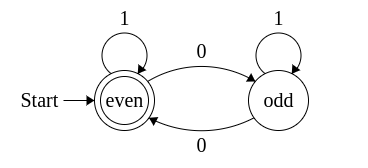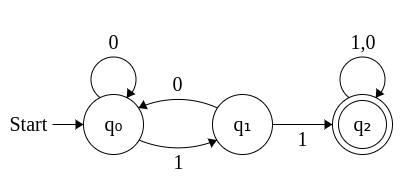# Vey.ie

Eoin Davey.
Problem solver/creator.
BSc. Computational Thinking.
Éireannach.

## The Problem

I recently encountered a problem which, while easy to define, was deceptively hard to solve

The problem went as follows. Given an integer N, and a substring S. How many binary strings of length N are there, that contain S?

So, for example, if asked How many binary strings of length 3 are there, that contain 11?, the answer would be 3.

011 110 and 111.

The 5 strings excluded being.

000, 001 010, 100 and 101

### Why not just run through all the strings?

A first thought might be to try all the strings of length N, and then check which strings contain S. It wouldn’t take long for a computer to generate all the strings if N is <= ~20, but after that you’ll find that every time N increases, the time required more than doubles.

This comes from the fact that there are 2^n binary strings of length N, so if asked to generate all the strings of length 100, you would need to generate over 1 billion billion billion strings. Or more visibly, over 1000000000000000000000000000000 strings

And then for each of those strings you would need to check if they contained S, which is another multiplier on how long it will take

In general this approach has complexity O(2^N N)

### How to check if a string contains S

If we take a step back from this problem, we might find something we can use. Lets consider, if we were given a string, how would we decide if it contains S.

You might try each position in the string, and check if there is a copy of S starting from that position, which for most strings is a simple and effective method

Someone who’s looking for a little more efficiency could employ an industry standard algorithm, such as KMP, which would work much faster

However there is a more interesting approach, Theoretical computer scientists will be familiar with concepts known as DFA’s. Often studied but seldom used in day to day problems. They are an important backbone to many useful things, such as regex.

### The DFA (Deterministic Finite Automaton)

For those unfamiliar with DFA’s, don’t let the name scare you off, they’re relatively simple machines that take in a string at one end and output YES or NO at the other end.

They’re constructed from a system of states and transitions, where each state has a transition saying where you should go next if you read a certain character. These are often represented as a system of circles and arrows, the circles represent the states, and the arrows the transitions.

The machine begins in the start state, and for each subsequent character it moves to the state indicated by the transition arrow. Whenever you reach the end of the string, you’ll finish in some state. Each state has a flag saying whether it is a YES (accept) state or a NO (reject) state. I’ll not go into depth on their structure here as only the basic idea is required for this problem.

For example here is a DFA to tell you if a binary string contains an even number of 0sYou start in the left hand state, if you read a 1 you stay where you are, and if you read a 0 you swap to the other state, this continues until the end of your string, If you are in the “accept” state, (the left state) the DFA accepts the string and you know the string has an even number of 0s, otherwise it rejects and your string has an odd number of 0s

A particularly interesting property of DFAs for us is that you can construct a DFA that can tell you if a string contains a certain substring S. And importantly, if the substring S has length M, then the DFA only needs M+1 states.

Here is the DFA to detect if a string contains “11”, as given by the above exampleIf you put in a string, and it ends in the last state, the string contains “11” As you can see the DFA has 3 states, which is 1 more than the length of “11”

I won’t cover the algorithm for constructing these DFA’s here, but it is included in the source code and is order O(M)

### Re-framing the question

Now that we have a directed graph representing the computation of whether a string contains a substring S, the question of “How many strings contain S” becomes “How many ways can we get from the start state of the graph to the end”.

Unfortunately the graph is directed, but it is not acyclic, so there are infinite ways to get the end node from the start node. But of course we’re not interested in every possible way to get from the start to the end, we’re only interested in how we can get there in exactly N steps.

We can reformulate our approach as a recursive function. If f(s,x) is the number of ways to get to the end state from the state s, in exactly x steps then intuitively you can formulate f(s,x) as

f(s,x) = f(d(s,0),x-1) + f(d(s,1),x-1)

where d(s,A) is the state you get by following the arrow for A from state s.

This follows from the fact that the number of ways to get to the end from a given state is the sum of the number of ways of getting to the end if you go down each edge, in our case each state has an edge for a 0 and an edge for a 1

The base case of our recursive function is

f(s,0) = 1 if we are at the end state and 0 otherwise

Our function now looks like this

int count(int u, int n){
if(n==0) // Base case, M is our final state
return u==M;
return count(dfa[u],n-1) + count(dfa[u],n-1);
}


### How fast is this approach?

Now that we have a formula to calculate, we can analyse it’s efficiency. If we take our formula at face value, each function evaluation results in 2 more calls, which results in a recursive tree of depth N. Therefore if we just try to evaluate the function written above we get an approach that is O(2^N), not much better than the brute force approach given above.

So have we gained anything be re-framing the question at all? Lets look closer at the function.

### Achieving polynomial time

The function takes 2 inputs, the state, and the number of remaining characters. If we have M states and N characters, there can be MxN possible different function calls, this is much less than 2^N for all inputs with more than 4 characters, so this means we must be recalculating the same functions multiple times.

For example, if our question is “How many strings of length 10 contain 10101 as a substring”, M = 6 and N = 10. Therefore we can have at most 60 different functions that need to be evaluated, but 2^10 = 1024, much more than 60, therefore we are recalculating functions we’ve already calculated at least 964 times. Can we eliminate these wasteful calls?

Notice that these functions are mathematically pure, i.e f(s,x) is always the same for the same s and x. So if we have already calculated the function, is there any reason to recalculate it again? Can’t we just save the answer and re-use it? Since we know it will be the same every time.

This is exactly what we can do, by employing an approach known as memoization or top-down dynamic programming we can avoid recalculating those functions every time by saving the result of the function into an array of answers, and before evaluating a function call we check the array to see if we’ve already calculated it. If we have we just re-use the answer we know is correct.

Here is our new improved function

int count(int u, int n){

return memo[u][n];

if(n==0) // Base case
return u==M;

return memo[u][n] = count(dfa[u],n-1) + count(dfa[u],n-1); // Calculate and store answer
}


So how fast is this algorithm now? Well you can now imagine it as a procedure that’s just filling in an N by M array, with each step taking constant time. So clearly it now only requires at most O(NM) steps, which importantly, is polynomial in time and memory

### Conclusion

By taking our question about strings and looking at it through a more abstract lens, we were able to apply methods from theory of computation, graph theory and algorithm optimisation to construct a polynomial time algorithm that is short, easily defined, and requires nothing more complex than arrays

This approach can of course be extended to strings of any alphabet, not just binary strings, the complexities gain an extra multiplicative factor of the size of the alphabet

I’ve attached the full program below, including algorithm for building the DFA

Code here